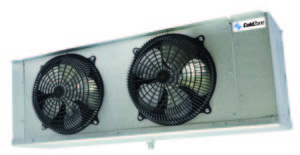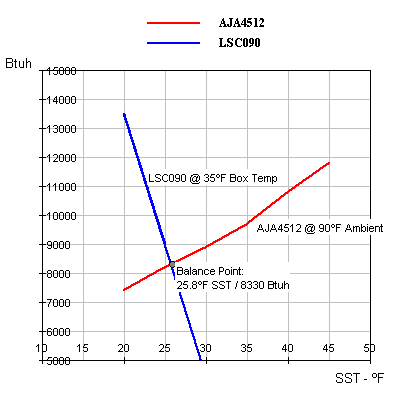﻿ HVACR Training Equipment Component Sizing Psychrometric Resource## Evaporator TD & System BalanceThis is taken from a past discussion in an HVAC-Talk thread about how to select a walk-in cooler evaporator so it will result in a suitable TD:

QUESTION:
Could someone explain to me how you size an evaporator to get a specific TD? I tried to understand the explanation in the Heatcraft Engineering Manual, but I just can’t seem to get it.

3. Determine what RH your product requires.

4. Find the proper coil TD range to achieve that RH from the Heatcraft Engineering Manual.

5. Select a condensing unit that will satisfy the box load at an evap temp equal to the box temp minus the TD.

6. Now…if you want to get real picky about it, you can plot out the system balance curve. This is a plot of Evap temp vs. Btuh which will show how the condensing unit capacity and evaporator capacity relate. Where the plots cross is where your system is balanced.

Clear as Mud?

QUESTION:

Definitely muddy…LOL

So let’s say I have a 10,000 BTU condenser at 20° SST and my evaporator is also 10,000 BTU. Box temp of 35°. That would be a 15° TD correct? So if I wanted to change the humidity in the box to 90%, which is 7-9° TD, could I just get a larger evaporator or would I have to get a smaller condenser?

Evaporators are typically rated at a 10ºF TD so in your example, the evaporator would have a capacity of 10,000 Btuh at a 10ºF TD or only 7000 Btuh at a 7ºF TD. If the 35ºF box load requires 10,000 then you need a bigger evap for the 7º TD. You need to select a coil with a nominal capacity of 10/7 x 10,000 or about 14,300 Btuh.

Then you need to select a condensing unit that has a capacity of 10,000 Btuh at your design evap temperature of +28ºF. (35º – 7ºF TD = 28ºF). The unit in your example would probably do close to 12,000 Btuh at +28ºF SST, so it’s too big.

Get a condensing unit catalog with the full performance charts and an evaporator catalog to study. Heatcraft’s site will have both.

Here’s a simple graph which demonstrates a basic compressor/evaporator balance:First plot the condensing unit capacity at various SST points taken straight from the catalog. Then plot the evaporator performance based on its specific capacity (Btuh/ºF TD) for a given design box temperature.

In this example the evaporator has a specific capacity of 900 Btuh/ºF TD, so at a 10ºF TD the SST would be 25ºF and the evap’s capacity would be 9000 Btuh. That’s one point on the graph. Do the same for 15ºF TD and 5ºF TD and you have a nice straight-line plot.

So it’s clear that where the two lines representing condensing unit and evaporator capacity cross is where the two capacities are the same…ie, that’s where the system will balance. The plot tells us the balance point is at 25.8°F SST.